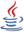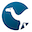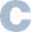# Expression Evaluation

Expressions are those things that we evaluate to produce values. Sounds like a pretty important thing to study.

## Expressions

At a fundamental level, programming can be viewed as nothing more than applying operators to operands. Operators can be built-in simple things like “`+`” or “`<`”, or can be built-in or user-defined functions, or can even introduce declarations, modify control flow, and cause side-effects.

LanguageExamples
```F                        -- invoke a zero-operand operator
F(X, Y, Z)               -- three operands for operator F
"+"(X, Y)                -- abbreviated X + Y
">"(X, Y)                -- abbreviated X > Y
```
C++
```f()
f(x, y, z)
operator+(x, y)          // abbreviated x + y
operator>(x, y)          // abbreviated x > y
```
Lisp
```(f)
(f x y z)
(+ x y)
(+ a b c d e)            /* Not just binary! */
(+ x (* y z))
```
Perl
```&f
f x, y, z                # Hmm, what does this mean?
f(x), y, z               # List of three things
f(x, y, z)               # Three-operand invocation
print 1, 3, sort 4, 2    # Gaaack
```
Standard ML
```f ()                     (* ALL ops have 1 operand *)
f x y z                  (* curried *)
f(x, y, z)               (* uncurried *)
op+(x, y)                (* same as x + y *)
```
Scala
```f                        // No parens is okay
f(x, y, z)               // Typical
x.+(y)                   // abbreviated x + y
df.format(date)          // abbreviated df format date
```

## Operators

### Operator Precedence

Motivation:

Does a • b ¶ c mean ((a • b) ¶ c) or does it mean (a • (b ¶ c)) ?

Higher precedence operators are applied first.

Language Issues:

• Maybe there's only one precedence level (e.g. APL, Smalltalk)
• Some languages have too many levels to memorize (e.g. C++, Perl)
• Some languages have nonsensical — or at least nonintuitive — precedence definitions (e.g. Pascal)
• Perl's distinction between named unary operators and other functions makes precedence impossible to distinguish by syntax only
• ML allows the programmer to change precedence at run time

### Operator Associativity

Motivation:

Does a • b • c mean ((a • b) • c) or does it mean (a • (b • c)) or are we not even allowed to write such a thing?

We speak of left-associativity, right-associativity, and non-associativity.

Observations:

• In Smalltalk, every operator is left-associative (and has equal precedence)
• In APL, every operator is right-associative (and has equal precedence)
• In many languages, arithmetic is usually left-associative, except for exponentiation
Exercise: Evaluate the expression `1-5-10` in Python, Smalltalk, and APL.
Exercise: Evaluate the expression `1-5*10` in Python, Smalltalk, and APL.
Exercise: Evaluate the expression `1*5-10` in Python, Smalltalk, and APL.
Exercise: Evaluate the expression `2**3**2` in Python.
Exercise: (Important) Evaluate the expression `-10<-5<-1` in JavaScript, Ruby, Ada, and Python, and explain in detail each of the four completely different behaviors!

### Operator Arity

The arity of an operator is the allowed number of operands. It can be fixed or variable. A variable arity operator is said to be variadic.

### Operator Fixity

Prefix, infix, postfix, overfix, underfix, outfix, ...

### Defining Operator Properties Through Syntax

We can encode precedence, associativity, arity, and fixity directly in the syntax, for example

```EXP   ⟶  ID  ":="  EXP  |  EXP1
EXP1  ⟶  (EXP1  LOGOP)?  EXP2
EXP2  ⟶  EXP3  (RELOP  EXP3)?
EXP3  ⟶  (EXP3  ADDOP)?  EXP4
EXP4  ⟶  (EXP4  MULOP)?  EXP5
EXP5  ⟶  EXP6  (EXPOP  EXP5)?
EXP6  ⟶  (UNARYOP  EXP6)?  |  EXP7
EXP7  ⟶  LITERAL  |  ID  |  FUNCALL  |  "(" EXP ")"
```

This implies

OperatorPrecedenceAssociativityArityFixity
Unary `-`
Unary `+`
HighestR1Prefix
`**` R2Infix
`* / %` L2Infix
`+ -` L2Infix
`< <= == != >= >` NO!2Infix
`and or` L2Infix
`:=`LowestR2Infix
Exercise: Make sure you really understand why relational operators are non-associative here.

## Evaluation Order

### Defined Order

Java forces a left-to-right ordering: `a-f(b)-c*d` means do the following, one after another:

 time ① `t0 ← f(b)` time ② `t1 ← a - t0` time ③ `t2 ← c * d` time ④ `t3 ← t1 - t2`

### Undefined Order

Most languages allow the evaluation order to be undefined so that the compiler can choose the best order it can. This is especially important for parallel architectures (multiprocessor or multicore).

For example, `a–f(b)–c*d` can be parallelized as

 time ① `t0 ← f(b)` time ② `t1 ← a - t0` ```t2 ← c * d ``` time ③ `t3 ← t1 - t2`

Also, `a:=b[i]; c:=a*2+d*3` can be done like this:

 time ① `t0 ← b + i` `t1 ← d * 3` time ② `a ← *t0` time ③ `t2 ← a * 2` time ④ `c ← t1 + t2`

Undefined ordering can lead to ambiguities or errors:

• Suppose g were a function which modified the global variable a as a side effect. Then the expression `f(a,g(b))` could yield different results depending on which order the arguments were evaluated.
• One might be tempted to do these two statements `a:=b; c:=d` in parallel, but if a and d are aliases of each other we can't.
• In languages that do saturated arithmetic or throw exceptions on arithmetic overflow, `(a+b)-c` might behave very differently from `(a-c)+b`, so a compiler shouldn’t rearrange operands unless it understands these semantics.
```\$ swift
1> let a: Int8 = 100
let a: Int8 = 100
2> let b: Int8 = 50
b: Int8 = 50
3> let c: Int8 = 40
c: Int8 = 40
4> (a - c) + b
\$R0: Int8 = 110
5> (a + b) - c
Execution interrupted. Enter code to recover and continue.
Enter LLDB commands to investigate (type :help for assistance.)```

### Short-Circuit Operators

The famous short-circuit logical operators are really control-flow mechanisms...

Expression Meaning Sometimes written as
```e1 andalso e2 e1 and then e2 e1 && e2``` If e1 if falsy, so is the whole and-expression, so you're done. Otherwise, the result is whatever e2 is. `if e1 then e2 else e1e1 ? e2 : e1`
```e1 orelse e2 e1 or else e2 e1 || e2``` If e1 if truthy, so is the whole or-expression, so you're done. Otherwise, the result is whatever e2 is. `if e1 then e1 else e2e1 ? e1 : e2`
Exercise: The languages in the C family handles these operators a bit differently from most other languages. Explain why.

Short-circuiting appears frequently in many programming idioms

• Null pointer check
```if (p != null && p.key == value) { ... }```
• Simulating a "default" parameter
```function f(x, y) {
y ||= 1;
...
}
```
• If something fails, take another action
```open(F, \$file) or die "Can't open \$file: \$!";```
• Continue with a second action only if something succeeds
```exists f && remove f```

## Side Effects

Note that evaluation order really only matters when side effects can occur (which is why immutability rocks!). Side effects are what occurs when a storage location is updated or when files or a database are read from or written to.

### Lvalues and Rvalues

Storage locations are denoted by lvalues. They are called lvalues because they can appear on the Left side of an assignment. Examples in C:

• `x`
• `x`
• `y.p()->q`
• `*(e1 + e2)`
• `*y`

An example of an rvalue is 500 (an integer literal).

Sometimes pretty complicated looking expressions can evaluate to lvalues! Examples:

```// C++, but not C
(x *= 10) += 7```
```# Perl
(\$x < 5 ? \$y : \$z) = 10;```
```# Perl
\$x = "dog";
\${\$x} = 2;
\$x = <STDIN>
chomp \$x;
\${\$x} = 2;```
```(* SML *)
val x = ref 0;
x := 3;
x := !x + 1;```

Sometimes, lvalues can be made read-only. Examples:

• Variables marked `const` in C, `let` in Swift, or `final` in Java.
• for-loop indices and in-parameters in Ada
• Variables outside of a function in Euclid and Turing

### Initialization vs. Assignment

Initialization and assignment are very different.

• Initialization creates a variable where none existed before
• Assignment updates the value of an existing variable

One language that makes the distinction explicit in code is C++. Examples:

```// C++ Initializations:
int x = 10;
int y(15);
int z(a + 5 / 2);
int w(x);
Point p(5, 12);
Point q = p;

// C++ Assignments:
x = 12;
y = x / 6;
q = p;
q = midpoint(p1, p2);```

Prefer initialization to assignment where possible. Here's a case where you must. Suppose we had a point class in C++:

```class Point {
public:
int x;
int y;
Point(int x1, int y1): x(x1), y(y1) {}
};```

Because we defined a constructor with parameters, we cannot ever define uninitialized points:

```Point p; // ILLEGAL

Point* p = new Point; // ILLEGAL

class Rectangle {
public:
Point corner1, corner2;
Rectangle(Point p, Point q) {  // ILLEGAL
corner1 = p;
corner2 = q;
}
};```

The Rectangle constructor failed because it is trying to initialize the fields to their default values and then assign them in the constructor body.... but there is NO default initializer for class Point. You MUST write the Rectangle constructor like this:

```class Rectangle {
public:
Point corner1, corner2;
Rectangle(Point p, Point q): corner1(p), corner2(q) {}
};```

## Lazy Evaluation

If expressions are only evaluated as-needed, or on demand, or only-if-needed, evaluation is said to be lazy. Otherwise it's eager.

```def first(x, y):
return x;

first(f(), g())
```

Under eager evaluation, both `f` and `g` are called, and the results of each are passed to `first`. Under lazy evaluation, only `f` gets called. In our example, suppose `f()` evaluates to 3 and `g()` to 5. Then:

EagerLazy
```first(f(), g())
= first(3, f())
= first(3, 5)
= 3
```
```first(f(), g())
= f()
= 3
```

In the cases where `g()` crashes or has other side effects, the difference between the two strategies can be a big deal.

Exercise: Do some research to see which languages are known for “being lazy.”

## Macros

A macro is code that gets expanded into new code which then gets compiled and run. In the simplest case, a macro gets expanded into source code, as in this example in C:

```#define area(r) (M_PI*(r)*(r))
double f(x) {
return 3 / area(x+10);
}```

but before the program is compiled, the C preprocessor expands the macro, producing:

```double f(x) {
return 3 / (M_PI*(x+10)*(x+10));
}```
Exercise: Suppose the above macro was (incorrectly) written as
`    #define area(r) M_PI*r*r;`
Show the expansion of `3/area(x+10)`.

More examples of C macros:

```#define MAX(x, y) ((x) > (y) ? (x) : (y))

#define mientras while

#define forever while(1)```
Exercise: Research (on the web is fine) the debate on whether C macros should be used as a last resort only.

C macros operate in source code. The macros of Lisp, Clojure, and Julia are much more sophisticated; these operate on abstract syntax trees. We’ll cover these when we get to metaprogramming.

Exercise: Research macros in Rust, Clojure and Julia.